# Math Modeling

Overview
Based upon our biological experimentation, we decided to build a temperature sensitive IFFL based upon the ts-CI system as a way to construct a decoder that could be tested for a variety of different dynamical inputs. To that end, we constructed a system of Ordinary Differential Equations (ODE) that would enable us to test the decoding performance of a ts-CI based IFFL system compared to a Naive tracker. We determined that the IFFL system is superior to the naive model, and is able to distinguish even small temporal inputs in many cases. Expanding on this we evaluated the effect of noisy inputs, finding that in all cases the ts-CI IFFL is more robust (less variable) than a naive model. We then evaluated how these properties vary when biologically tractable parameter of degradation rate is changed. Lastly, we also created several other models for the various portions of our projects, which can be seen here.
Distinguishability
To assess if Zhang’s findings apply to our system, we first needed to come up with a way to measure the ability of the decoding circuits to distinguish different temporal inputs. We achieved this by creating a naive model that is a simple tracker of gene expression (i.e. an activatable gene with no additional regulation). Then, to compare the ability of the decoding circuit to the naive tracker, we gave both systems two identical pairs of temporally distinct inputs and then compared their distinguishability by determining which circuit gave more distinct outputs in the amplitude domain. In our initial tests, we found that for some inputs the IFFL performs superiorly to the naive circuit (Fig 1 left) whereas for others, the naive circuit performed marginally better (Fig 1 right).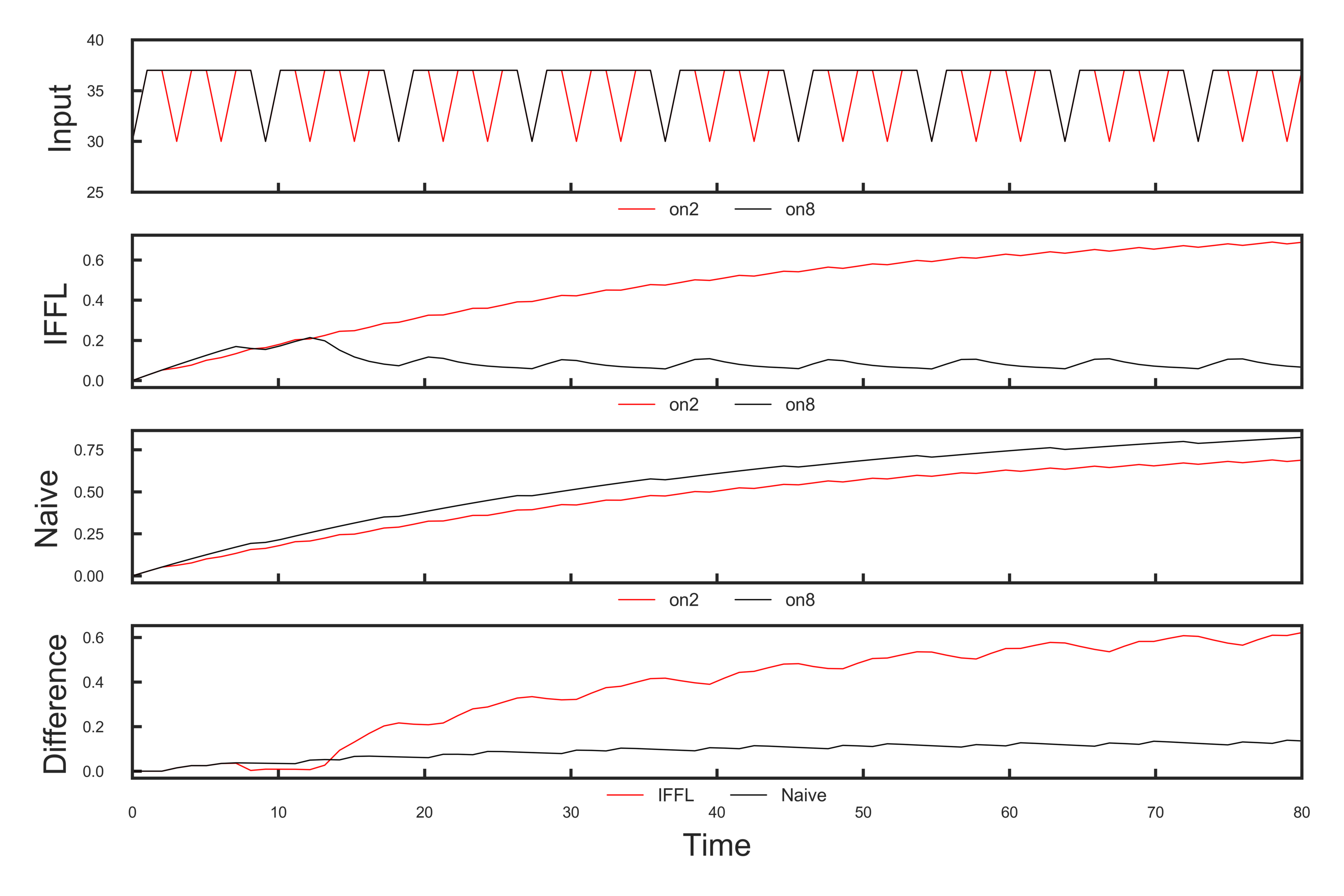Figure. 1: Left: off = 1, on1 = 2, on2 = 8. Input (top); Output for IFFL; Output for Naive; Difference in outputs (bottom). Right: off = 10, on1 = 2, on2 = 8. Input (top); Output for IFFL; Output for Naive; Difference in outputs (bottom).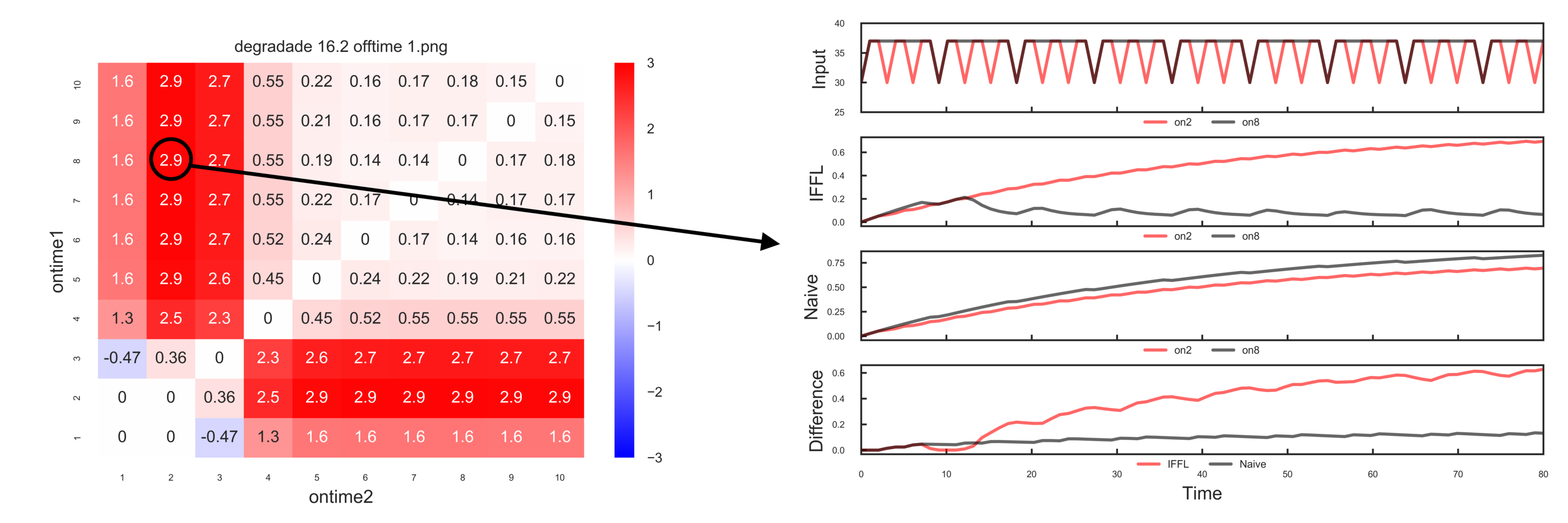Figure. 2: First two graphs represent two different distinct inputs of different on times with fixed off time. Based on these inputs, expression concentrations are calculated for both IFFL and Naive, with their differences represented as a vector, and the final values being shown in the heat map below. The bottom right graph shows general behavior of the systems at two different on times: The second subplot showing expression by IFFL and the third by Naive. The last subplot shows the concentration differences between those two distinct inputs as a function of time. Higher difference in the last subplot indicates better decoding ability.
##### Result I. Effect of On/Off time
IFFL is always better at decoding in the domain range where the on time for the input is long enough to trigger the transitional behavior of the reporter from stair-case to pulse-like steady state (see Figure. 2, IFFL subplot). This is due to the induced degradation rate of m-Scarlet by mf-Lon as the protease passes its half-activation threshold, which is triggered by sustained on time. When it comes to observing vector difference between two similarly closed on times (but not long enough to observe the transition behavior in reporter), IFFL and Naive performed similarly at distinguishing these temporal structures. In general, IFFL allows a wider range of distinguishable time-domain inputs relative to the Naive system.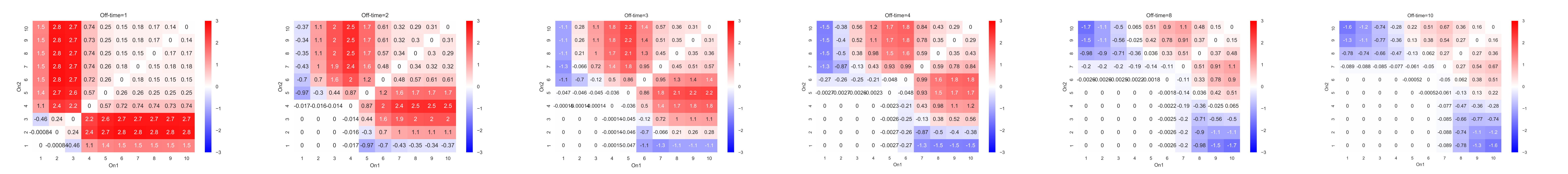Figure 4: The combined graphs of when off time=1, 2, 3, 4, 8 and 10. The trend is illustrated: as off time becomes longer, IFFL gradually lose its ability to distinguish input signals due to shorter on time relative to total period. Notice the heat map's symmetry along the diagonal. (Reproduced from Fig.1 using the same degradation rate)
##### Result II. Effects of Induced Degradation Rate
We also observed the heat map with varying induced-degradation rate of m-Scarlet by mf-Lon, dMS. According to Zhang's paper, when dMS is sufficiently low, the ability for IFFL to distinguish different temporal structure diminishes. Therefore, as dMS increases, the time input distinguishing ability of IFFL increases.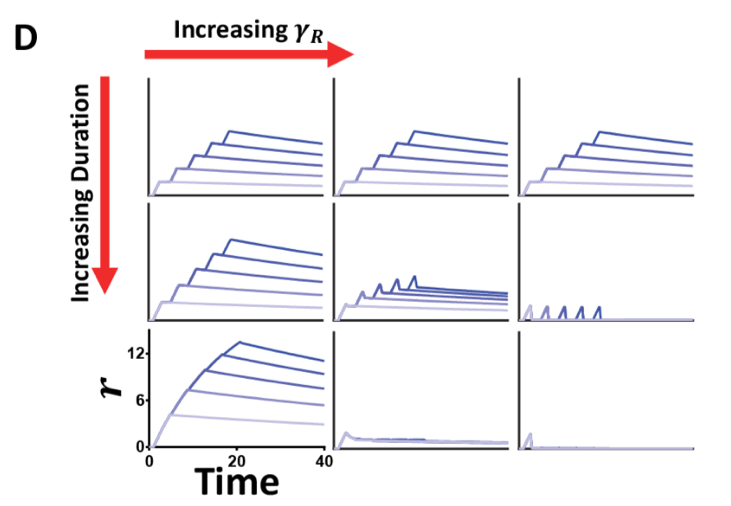Figure 5: Effect of varying dMS and duration on time courses. Time courses with different number of pulses demonstrate the range in the distinguishing capability for a changing dMS. As dMS increases, the ability to differentiate pulsing and more sustained inputs increase.
By observing values on heat maps, we reached the conclusion that increase in induced degradation rate leads to better performance of IFFL in general. For some regions, when IFFL is extraordinarily superior to Naive system (for example, when difference = 2.5), increase in dMS yields even better decoding performance; for some regions, when IFFL is moderately better at decoding input than the Naive system, increase in dMS lowers IFFL's ability to decode input structure.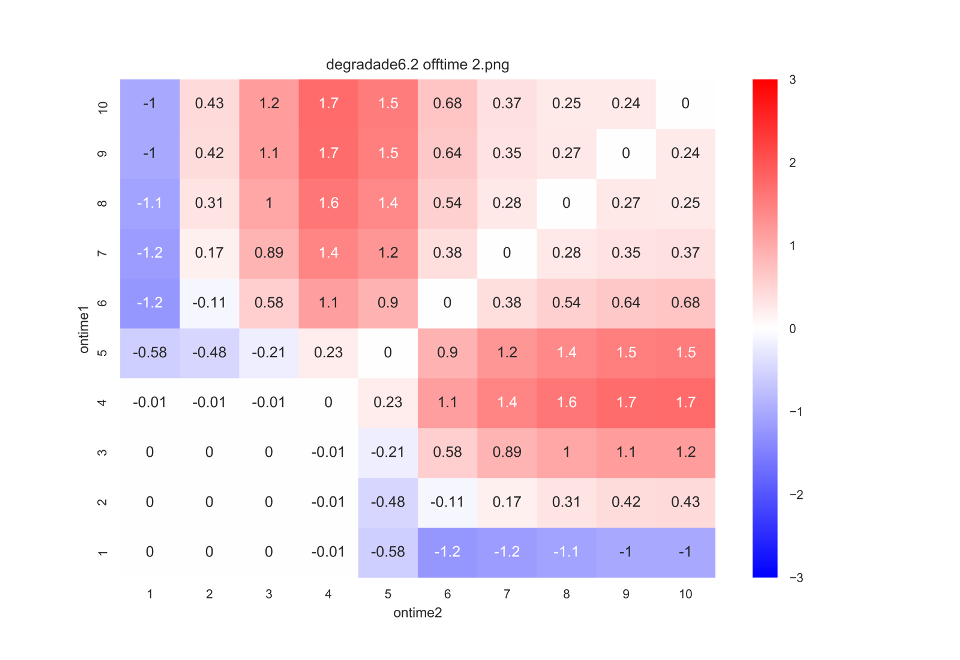Figure 6: Varying induced degradation rate affects IFFL's decoding performance. (Left) when dMS=6.2x, the highest vector difference is 1.7, and lowest value is -1.2. (Right) when dMS is 26.2x, the highest red value is 2.8, the lowest value is -0.94. We could see that IFFL's performance improved in general. The column corresponds to on2=4 has much higher value compared with the same column when dMS = 6.2x. In addition, the column for On2=1 when dMS = 26.2x also has much higher value as the saturation of blue areas decrease. However, for some cases where IFFL is just moderately better at decoding input structure than Naive, IFFL's performance becomes worse, observing that values of right-side columns decrease.
Noise Robustness
With IFFL's ability to detect small variations in temporal structure from the input, we would expect that the system would be susceptible to noise. To test this hypothesis, we performed the following numerical testing for both IFFL and Naive model and compare the variance as direct translation to noise response. Gaussian noise with average value 0 and standard deviation of 0.1 was applied to the concentration of CI: Start with fixed value for off-on ratio, calculate all concentration expressions of m-Scarlet within a specific parameter region of m-Scarlet production rate. Variance is then taken for that specific off-on ratio, then on ratio is incremented, then off ratio is incremented as on ratio resets. Each variance for respective off-on ratio is the average variance of concentration expression as function for varying parameter value range of m-Scarlet production rate.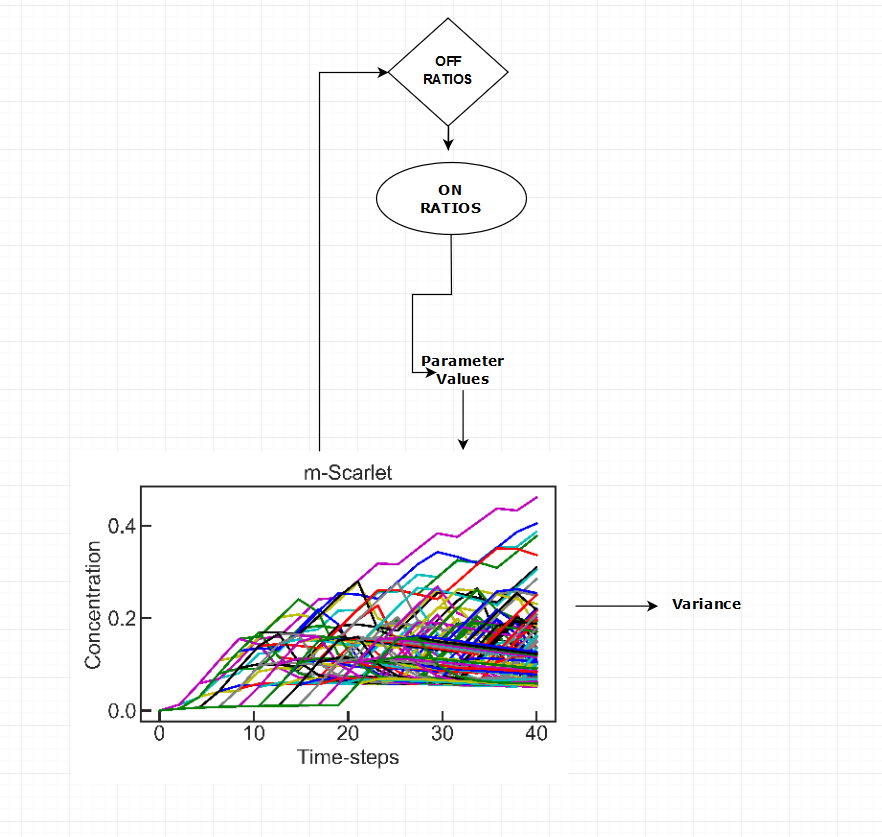Figure. 7: Scheme of Variance Test as Direct Translation to Noise Response for both IFFL and Naive.
We believe that this is the appropriate method to assess the system's ability to respond to noise. Production rate of m-Scarlet was selected to be the changing parameter region since this is analogous to either changing values for degradation rate of mf-Lon or the induced degradation rate of m-Scarlet by the protease. The method not only covered output expressions in wide range of parameter regions, but it also guaranteed to illustrate output's behavior across all possible range of structural inputs.
##### Result III. Robustness to Noise
The demonstration of noise damping property of IFFL can be explained through proportionality effect of mf-Lon. Even with noisy inputs, mf-Lon is produced accordingly to weaken the effect of stochastic expressions seen in m-Scarlet. In the case of naive’s high variance, result shows very consistent noise response through all combination of off and on ratios. Regardless, IFFL is exponentially more robust to noise than naive.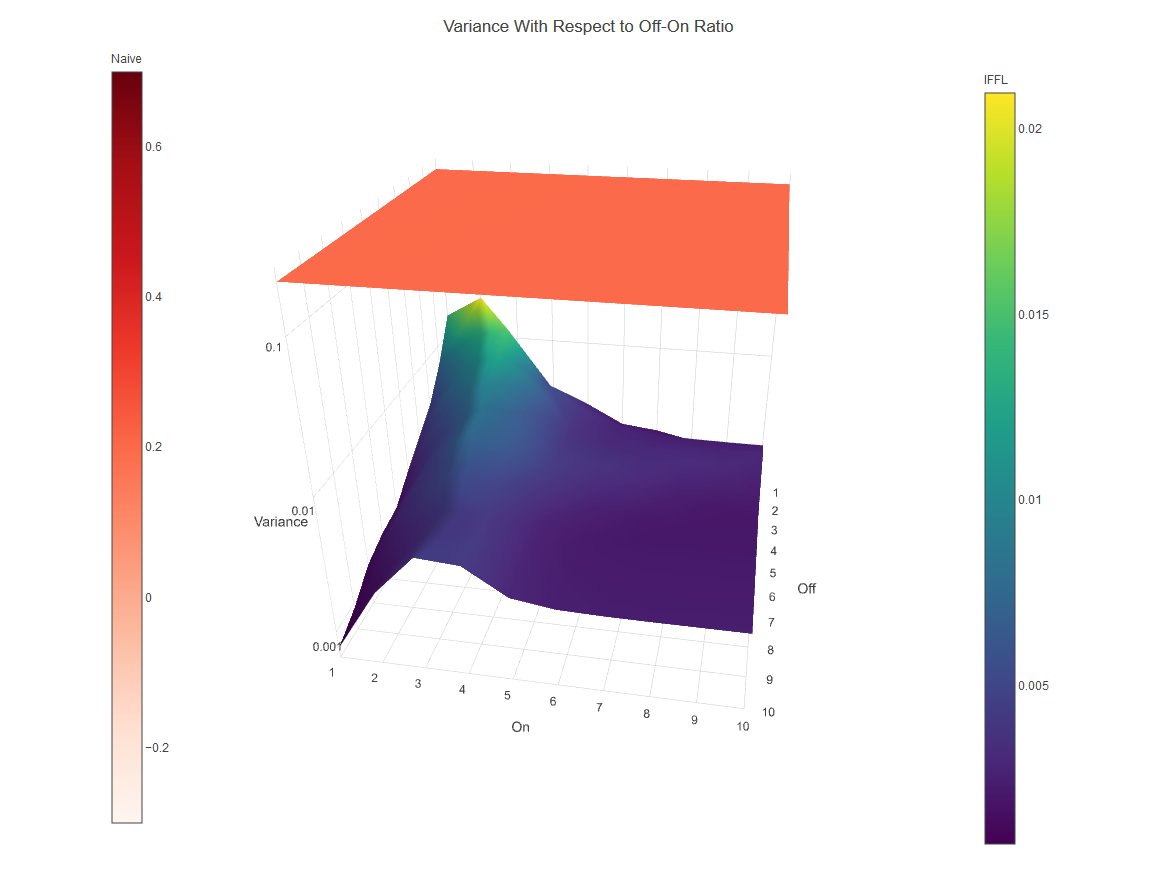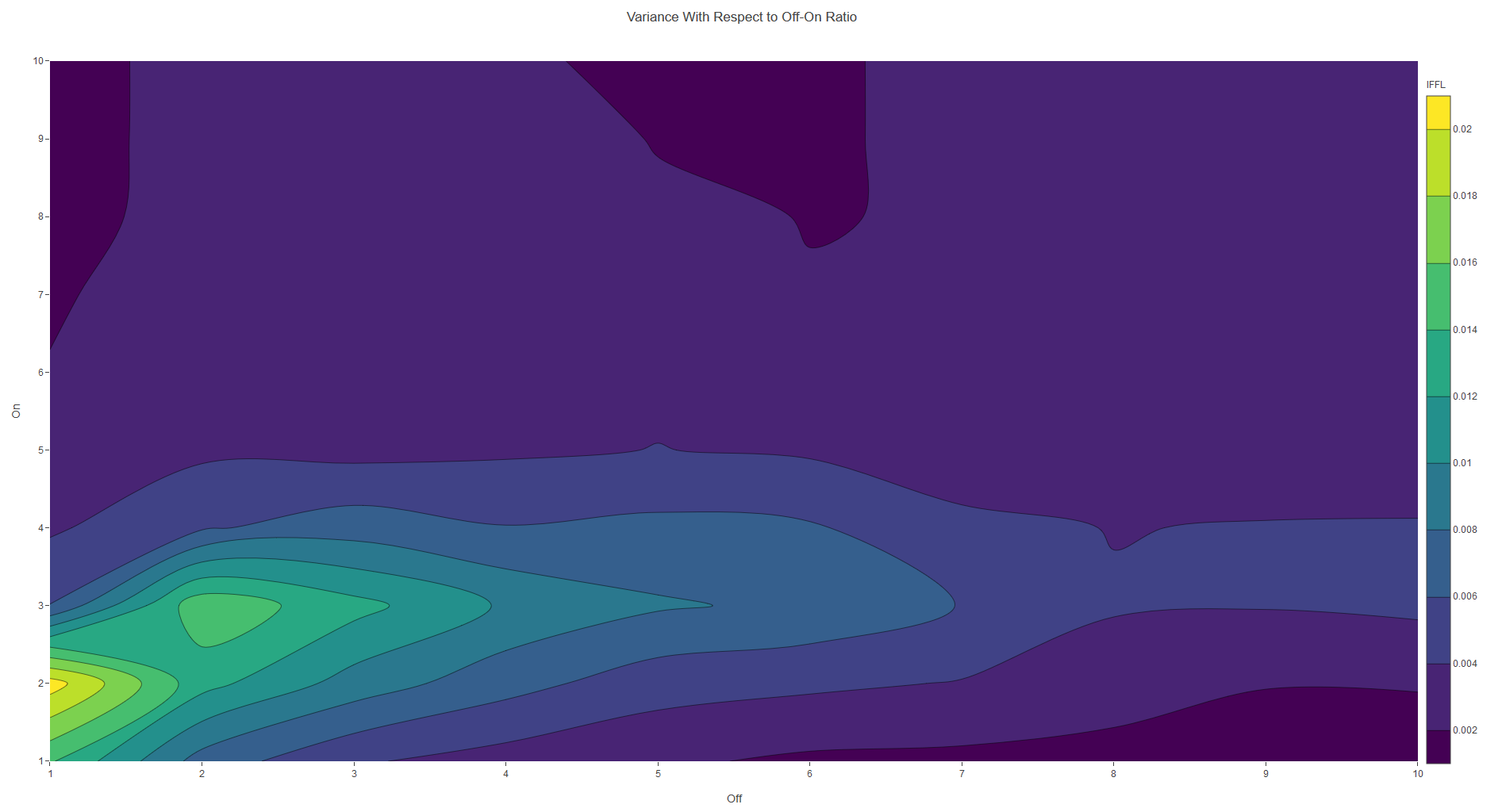Discussion
The goal of this model was to demonstrate the effectiveness of IFFL at processing information in time-domain inputs relative to the performance of its alternative, Naive system. Through this lens, aspects such as input distinguishing abilities and sensitivity to noise were examined through numerical computation and analysis, and the results indicate succinct distinction in IFFL's performance.
When structural inputs are widely different, it is obvious to detect such changes from the output expression in any arbitrary circuits. However, the ability to identify a wide range of temporal strucutures in terms of on and off ratios is the appealing characteristic of IFFL. Moreover, computational result revealing the motif's high fidelity in responding to noisy input ensures the functionality of the circuit compared to its alternative. With these findings, we hope future iGEM teams could built upon this foundation to go further on the insight of how natural systems encode time-domain information, and based on this knowledge, implement future synbio projects useful in this track but be aware that your signal of interest should encode information in the time-domain with appropriate difference between off and on times and a high induced degradation rate should be implemented.
Reference
 Zhang C, Tsoi R, Wu F, You L (2016) Processing Oscillatory Signals by Incoherent Feedforward Loops. PLoS Comput Biol 12(9): e1005101.